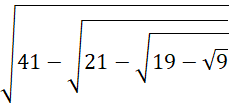# Quiz Discussion

If $$\sqrt 5 = 2.236{ \text{,}}$$   then the value of $$\frac{1}{{\sqrt 5 }}$$ is = ?

Course Name: Quantitative Aptitude

• 1] 0.367
• 2] 0.447
• 3] 0.745
• 4] None of these
##### Solution
No Solution Present Yet

#### Top 5 Similar Quiz - Based On AI&ML

Quiz Recommendation System API Link - https://fresherbell-quiz-api.herokuapp.com/fresherbell_quiz_api

# Quiz
1
Discuss

$$\sqrt {176 + \sqrt {2401} }$$    is equal to = ?

• 1] 14
• 2] 15
• 3] 18
• 4] 24
##### Solution
2
Discuss

What is the least number which should be subtracted 0.000326 in order to make it a perfect square = ?

• 1] 0.000002
• 2] 0.000004
• 3] 0.02
• 4] 0.04
##### Solution
3
Discuss

1250 oranges were distributed among a group of girls of a class. Each girl got twice as many oranges as the number of girls in that group. The number of girls in the group was = ?

• 1] 25
• 2] 45
• 3] 50
• 4] 100
##### Solution
4
Discuss

Evaluate -• 1]

3

• 2]

5

• 3]

6

• 4]

6.4

##### Solution
5
Discuss

The square root of $$\frac{{{{\left( {0.75} \right)}^3}}}{{1 - 0.75}} { \text{ + }}\left[ {0.75 + {{\left( {0.75} \right)}^2} + 1} \right]$$    is = ?

• 1] 1
• 2] 2
• 3] 3
• 4] 4
##### Solution
6
Discuss

$${1.5^2} \times \sqrt {0.0225} = ?$$

• 1] 0.0375
• 2] 0.3375
• 3] 3.275
• 4] 32.75
##### Solution
7
Discuss

If √x+x/y  = x√x/y  where x and y are positive real numbers, then y is equal to ?

• 1]

x + 1

• 2]

x - 1

• 3]

x2 + 1

• 4]

x2 - 1

##### Solution
8
Discuss

The number of trees in each row of a garden is equal to the total number of rows in the garden. After 111 trees have been uprooted in a storm, there remain 10914 trees in the garden. The number of rows of trees in the garden is = ?

• 1] 100
• 2] 105
• 3] 115
• 4] 125
##### Solution
9
Discuss

$${\left( {15} \right)^2} + {\left( {18} \right)^2} - 20 = \sqrt ?$$

• 1] 22
• 2] 23
• 3] 529
• 4] 279841
• 5] None of these
##### Solution
10
Discuss

The least number by which 294 must be multiplied to make it a perfect square, is = ?

• 1] 2
• 2] 3
• 3] 6
• 4] 24
# Quiz